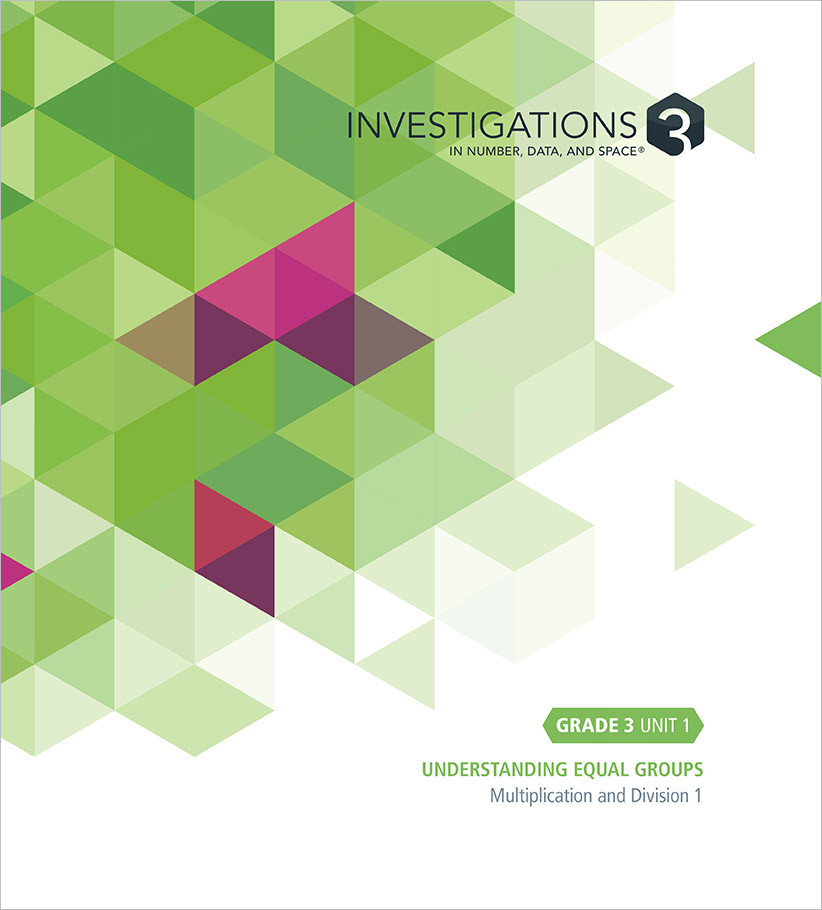Select Page

#### Unit 1: Understanding Equal Groups (23 Sessions)

##### Multiplication and Division 1

This unit focuses on understanding the meaning of multiplication, modeling multiplication using arrays, and understanding the inverse relationship between multiplication and division. Students make lists of things that come in groups and use them to write multiplication and division story problems, and equations to match. Multiples and arrays are introduced as two ways to represent multiplication. Students use arrays, and known facts, to develop fluency with the multiplication facts, and are assessed on those that involve multiplying numbers to 10 by 1, 2, 5 and 10. They find factors of numbers up to 100, and examine the relationship between multiplication and division.

• Investigation 1: Things That Come in Groups (4 Sessions)
• Investigation 2: Skip Counting and 100 Charts (6 Sessions)
• Investigation 3: Arrays (7 Sessions)
• Investigation 4: Understanding Division (6 Sessions)

#### Unit 2: Graphs and Line Plots (15 Sessions)

##### Modeling with Data

This unit focuses on using bar graphs, pictographs, and line plots to represent, describe, and compare categorical and numerical data. They solve one- and two-step “how many more” and “how many less” problems using information presented in the graphs. Students also generate measurement data in inches, half-inches, feet, and yards.

• Investigation 1: Modeling with Data (9 Sessions)
• Investigation 2: Collecting, Representing, and Analyzing Measurement Data (6 Sessions)

#### Unit 3: Travel Stories and Collections (25 Sessions)

##### Addition, Subtraction, and the Number System 1

This unit focuses on understanding and extending knowledge of place value and the number system to 1,000, and adding and subtracting accurately and efficiently. Students use a place value context (the Sticker Station) to represent numbers as hundreds, tens, and ones, and find equivalent ways to use 100s, 10s, and 1s to represent a given number. They construct and locate numbers on a 1,000 Chart. They also develop strategies for adding and subtracting 2-digit and 3-digit numbers with sums and differences to 400. Students encounter a variety of different addition and subtraction problems types.

• Investigation 1: Working with 100 (5 Sessions)
• Investigation 2: Building 1,000 (4 Sessions)
• Investigation 3: Addition (5 Sessions)
• Investigation 4: Finding the Difference (5 Sessions)
• Investigation 5: Subtraction Stories (6 Sessions)

#### Unit 4: Perimeter, Area, and Polygons (17 Sessions)

##### 2-D Geometry and Measurement

This unit focuses on understanding and finding perimeter and area using standard units of measurement, and on classifying 2-D figures. Students use standard measurement tools to measure the length of objects and the distance around 2-dimensional figures (perimeter). They use square units to measure the amount of space a given object covers (area). Students build on work connecting arrays and multiplication, and find area by multiplying length and width. They also recognize area as additive: a shape can be composed and its area found by adding the areas of the parts. Students look at rectangles that have the same area and different perimeters, and the same perimeter and different areas. Students also identify attributes of triangles and quadrilaterals, and explore different categories of quadrilaterals.

• Investigation 1: Linear Measurement (5 Sessions)
• Investigation 2: Understanding and Finding Area (7 Sessions)
• Investigation 3: Triangles and Quadrilaterals (5 Sessions)

#### Unit 5: Cube Patterns, Arrays, and Multiples of 10 (17 Sessions)

##### Multiplication and Division 2

This unit focuses on the meaning and structure of multiplication and division and the relationship between them, solving multiplication and division problems, multiplying by multiples of 10, and learning the remaining multiplication facts to 10 × 10. Students use connecting cubes to identify and analyze arithmetic patterns and the relationship between multiplication and division. Problems about multiplying single-digit numbers by multiples of 10 and multi-step problems are set in the context of a toy factory.

• Investigation 1: Relationship between Multiplication and Division (5 Sessions)
• Investigation 2: Solving Multiplication and Division Problems (6 Sessions)
• Investigation 3: Multiplying by Multiples of 10 (6 Sessions)

#### Unit 6: Fair Shares and Fractions on Number Lines (13 Sessions)

##### Fractions

This unit focuses on understanding the meaning of fractions as numbers and as equal parts of a whole; reasoning about equivalent fractions; comparing fractions; and using notation to model fractions and fraction relationships. Students build their knowledge of fractions and fraction equivalents as they represent and compare fractional quantities, including fractions greater than one, with pattern blocks and on the number line.

• Investigation 1: Sharing Brownies (8 Sessions)
• Investigation 2: Many Ways to Make a Share (5 Sessions)

#### Unit 7: How Many Miles? (18 Sessions)

##### Addition, Subtraction, and the Number System 2

This unit focuses on understanding the operations of addition and subtraction, and adding and subtracting fluently. Students add multiples of 10 and 100 to, and subtract them from, 3-digit numbers. They use multiples of 100 as landmarks as they solve addition and subtraction problems with 3-digit numbers, including problems that involve liquid volume and mass. Students share, discuss, and compare different strategies for addition and subtraction.

• Investigation 1: Numbers in the Hundreds (7 Sessions)
• Investigation 2: Addition Strategies (5 Sessions)
• Investigation 3: Subtraction (6 Sessions)

#### Unit 8: Larger Numbers and Multi-Step Problems (16 Sessions)

##### Multiplication and Division 3

This unit focuses on solving multiplication and division problems, learning the division facts, identifying arithmetic patterns, and solving multi-step problems. Students develop strategies to solve multiplication and division problems, including problems with remainders. Stories about children who receive a certain number of marbles each night presents a context for multi-step problems. They use representations and tables to compare different situations and develop a rule for describing the relationship between the number of days and the total number of marbles.

• Investigation 1: Solving Division Problems (6 Sessions)
• Investigation 2: Solving Multiplication and Division Problems (5 Sessions)
• Investigation 3: Solving Multi-Step Problems (5 Sessions)